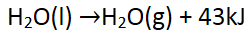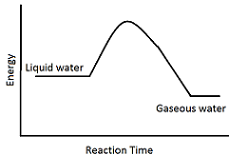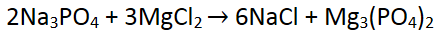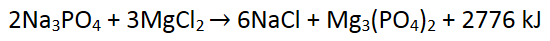# Thermochemical Equations

Instructor: Laura Foist

Laura has a Masters of Science in Food Science and Human Nutrition and has taught college Science.

In this lesson we will learn about thermochemical equations and how we use them to tell what happens to energy in equations and if the reaction is endothermic or exothermic.

## Thermochemistry and Reactions

If energy is always conserved then where does the energy come from to make reactions happen? This addition (or loss) of energy isn't typically included in the reaction. But, when we write a thermochemical equation we include this energy transfer. According to Newton's Law of Conservation of Energy, the total energy of a system is always conserved (stays the same).

Energy can be transferred through light, movement, and heat. In a chemical reaction, energy is either used or released. If energy is released the reaction is exothermic (energy exits). If energy is transferred in (energy enters) then it is endothermic.

The energy transfer in chemical equations can be measured by measuring the heat transfer. Bonds between atoms hold energy in them. When those bonds are broken, that energy is released, often in the form of heat. A calorimeter can be used to measure the amount of heat released when the bonds are broken. This is done by burning the compound until it is individual atoms to find the amount of energy held in the bonds. We are then able to determine how much energy is in a compound. The amount of energy held in a compound is called the enthalpy of combustion or formation. These enthalpies have been recorded in charts.

## Physical Change Calculations

To calculate how much energy has been transferred in a reaction we need to know the starting and ending energy. We look at the total energy of all the reactants and the total energy of all the products and determine the difference (products minus reactants).

Even physical changes can have a change in energy. An example is water going from a liquid to a gas. The total energy in liquid water is -285 kJ/mol, and the total energy in gaseous water is -242 kJ/mol. If we break all the bonds in liquid water it releases 285 kJ/mol, but if we break all of the bonds in gaseous water it only releases 242 kJ/mol. This means that when water is changed from a liquid to a gas there is a release of energy into the environment of 43 kJ/mol (-285 - (-242) = -43). This makes sense because the bonds in gaseous water are not as tightly ordered so they do not hold as much energy in them as those in liquid water. The properly balanced thermochemical equation includes the delta -43kJ/mol:When we go backward in an equation the sign of the energy changes. To go from gaseous water to liquid water we need to put in 43 kJ/mol of energy. We need to add heat or energy.

When we look at the change in energy we are looking at the difference from the beginning to the end. Often somewhere in the middle energy still needs to be put in, called activation energy, but once the exothermic reaction is done it is at a lower energy state.## Exothermic Reaction Calculations

Let's look at another example, this time where actual bonds are rearranging.If we are given the information that the heat of formation for each of the compounds is as follows:

• Sodium Phosphate: -1936 kJ/mol
• Magnesium Chloride: -642 kJ/mol
• Sodium Chloride: -411 kJ/mol
• Magnesium phosphate: -466 kJ/mol

Adding up the total energy in each of the reactants gives:

(2 * -1936) + (3 * -642) = -5798.

There is a total of -5798 kJ/mol of energy in the reactants.

The total energy in the products is:

(6 * -411) +(-466) = -2,932.

There is -2932 kJ/mol of energy in the products.

Since -5708 - (-2932) = -2776, there was a release of 2776 kJ/mol of energy, probably in the form of heat.

The negative sign means that energy was released. Notice that we multiplied each compound by the stoichiometric coefficient, which is the number of moles of each compound in the balanced chemical equation. This is because the heat of combustion is given per mol, so if the reaction has two moles of sodium phosphate then we need to multiply the heat of combustion by two.To unlock this lesson you must be a Study.com Member.

### Register to view this lesson

Are you a student or a teacher?

### Unlock Your Education

#### See for yourself why 30 million people use Study.com

##### Become a Study.com member and start learning now.
Back
What teachers are saying about Study.com

### Earning College Credit

Did you know… We have over 160 college courses that prepare you to earn credit by exam that is accepted by over 1,500 colleges and universities. You can test out of the first two years of college and save thousands off your degree. Anyone can earn credit-by-exam regardless of age or education level.